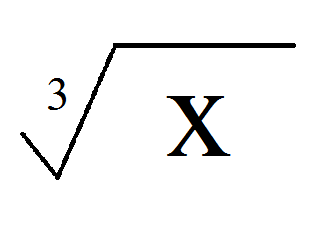# Simple Math Post: Linear Equations *1 variable*

Hi,

There are some students who need a lot of help with solving linear equations. As the students enter 7th grade, they have to deal with a lot of equations and how to solve them. Actually, it is easier than it looks!

LINEAR EQUATIONS

Now, you have most probably got to see an equation. It is full of x’s, y’s,÷’s, -‘s, +’s and ×’s. But, as I said before, it is easier as it looks. An equation is an expression which has = signs to balance each side. An equation is more like a balancing machine where if 2 mangoes is equal to 100 kg, then you divide both sides by two to get the weight of one mango. 100÷2=50 kg.In this equation, we have fractions. This can also be written as-

(x÷5)+1/3=4/3

We solve.

x/5 + 1/3 (-1/3)=4/3 (- 1/3)=3/3

x/5=1

x=1×5=5

As you see that in this equation, an extra 1/3 is added to x/5. We must make 1/3 to zero so that we only get x/5. But, we have to balance both equations which can be seen. By subtracting 1/3 from both sides, we get x/5 on the LHS (Left Hand Side) and,

4/3-1/3=3/3

on the RHS (Right Hand Side). NOW, we have

x/5=1

How do we get x? To get x, you have to multiply by 5 because multiplication is the opposite of division.

x=1×5=5

While solving linear equations, we look at inverse operations. We take the conjugate (/ˈkɒndʒʊɡeɪt/) or inverse operation of the number.Now you can notice a difference between +, – ,and ×, ÷. When you multiply a fraction

x/d×d=x/1

you always get x/1, the same case when you

x × (d÷d)= x × 1

But, if you have an addition-

x+d

When, you subtract from this, you get

x+d-d=x+0

And,

x-d+d=x-0

This is a very useful property you need to know.

If you have a number and some equation y, and

a(y)=l

First, divide by a.

y=l/a

Then, solve.

The inverse of the operation called root is the exponent.IS THE INVERSE OF.IS THE INVERSE OFWhen you have the same variable or unknown on both sides of the equation-

x-10=2x+29

But, they have different coefficients. So, to remove one x,

x-10-x=2x+29-x

-10=x+29

and then solve for x-

-10-29=x=-39

HOTS Question

1. Solve for x-

5(x-3)=10

2. Solve for a-

10-3(a+13)=a-9

3. Solve for y-

9y+1=6y

4. Solve for x-

8(10+x)-6=10